# how many moles are present in 0.00339g of LiF?

how many moles are present in 0.00339g of LiF?

Given mass of LiF =0.00339g

Mass of one mole of LiF (molar mass)=25.939 g/mol

Number of moles present = given mass/molar mass =0.00339g/25.939g/mol =1.30×10-4 mol of LiF

Thank you

#### Earn Coin

Coins can be redeemed for fabulous gifts.

Similar Homework Help Questions
• ### How many moles of LiF are present in a 42.0 g sample? • Your answer should...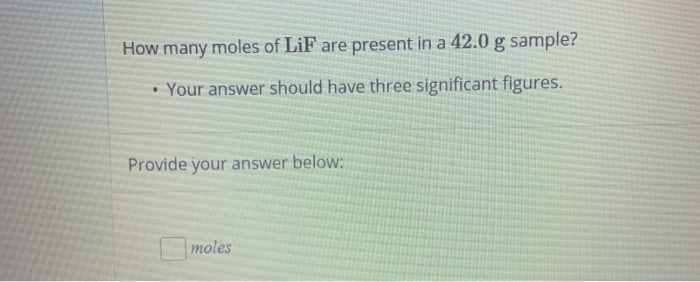How many moles of LiF are present in a 42.0 g sample? • Your answer should have three significant figures. Provide your answer below: moles

• ### How many milliliters of a 0.222 M LiF solution contain 0.444 moles of LiF?

How many milliliters of a 0.222 M LiF solution contain 0.444 moles of LiF?

• ### how many moles of water, H2O, are present in 25.0 g H2O? How many moles of...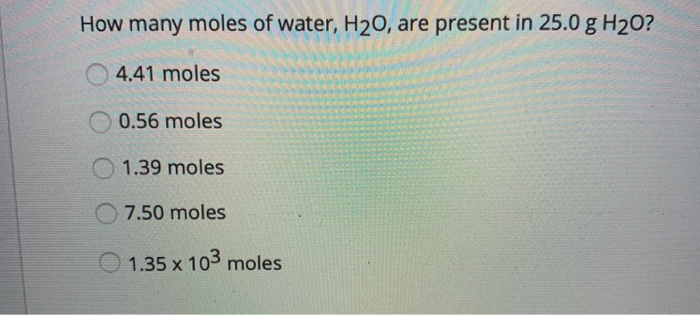how many moles of water, H2O, are present in 25.0 g H2O? How many moles of water, H20, are present in 25.0 g H20? 4.41 moles 0.56 moles O 1.39 moles 7.50 moles 1.35 x 103 moles

• ### #6: How many moles of sodium chloride are present in 9.29 g? How many moles of...

#6: How many moles of sodium chloride are present in 9.29 g? How many moles of sodium are present in the sample? please show work

• ### Determine how many moles of HOAc and how many moles of OAc^- are present 50. mL...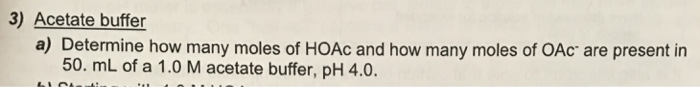Determine how many moles of HOAc and how many moles of OAc^- are present 50. mL of a 1.0 M acetate buffer, pH 4.0.

• ### a. How many moles of xenon trioxide are present in 4.95 x 1022 molecules of this...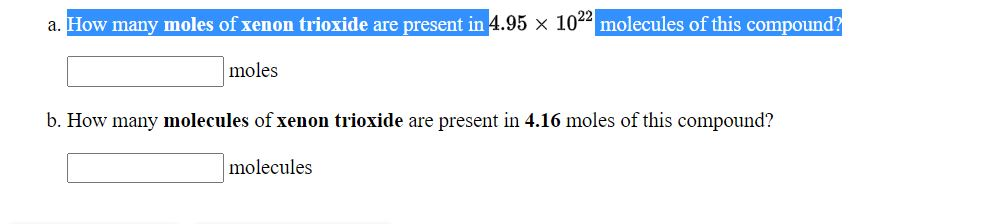a. How many moles of xenon trioxide are present in 4.95 x 1022 molecules of this compound? moles b. How many molecules of xenon trioxide are present in 4.16 moles of this compound? molecules a. How many moles of nitrogen dioxide are present in 2.77 x 1022 molecules of this compound? moles b. How many molecules of nitrogen dioxide are present in 1.31 moles of this compound? molecules a. How many molecules of tetraphosphorus decaoxide are present in 2.05 moles...

• ### how many moles of KOH are present

how many moles of KOH are present in 10.4 mL of 0.873 M KOH?

• ### how many molecules of tetraphosphorus decaoxide are present in 4.31 moles of this compound ? and...

how many molecules of tetraphosphorus decaoxide are present in 4.31 moles of this compound ? and how many moles of tetraphosphorus decaoxide are present in 1.07x10^22 molecules of this compound ?

• ### Part A How many moles of sulfur are present in 3.5 moles of Al2(SO4)3? Express the...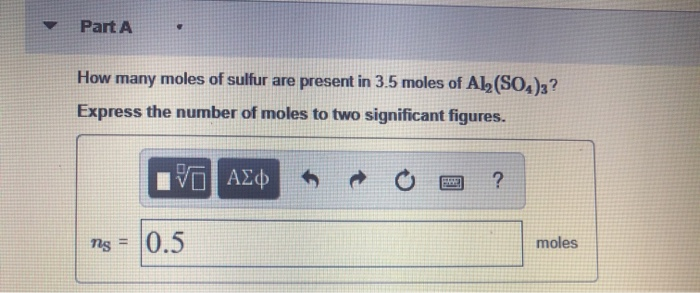Part A How many moles of sulfur are present in 3.5 moles of Al2(SO4)3? Express the number of moles to two significant figures. V AX“ o ? 0.5 moles Part B How many moles of aluminum ions are present in 0.36 moles of Al(SO4)3? Express the number of moles to two significant figures. VO AEM O O ? TAI = 0.6 mol Submit Previous Answers Request Answer Part How many moles of sulfate ions (S0,2 - ) are present in...

• ### how many moles of MgCl ny moles of MgCl2 are present in 60.0 mL of a...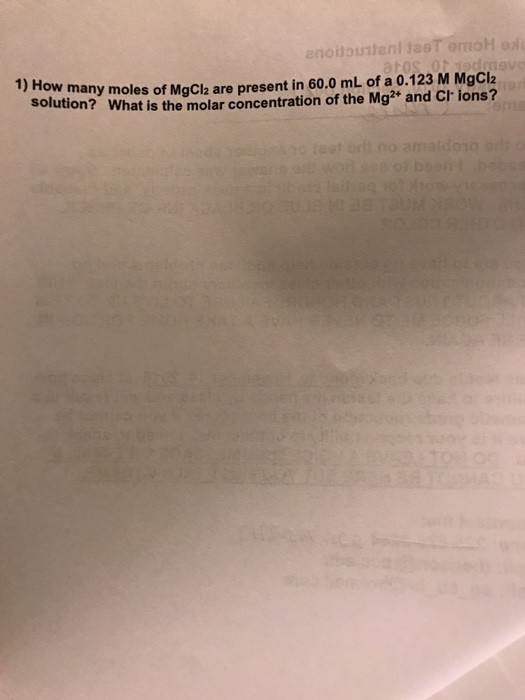how many moles of MgCl ny moles of MgCl2 are present in 60.0 mL of a 0.123 M MgCl2 What is the molar concentration of the Mg2+ and Cr ions? 1) How many mo solution? What Solution?